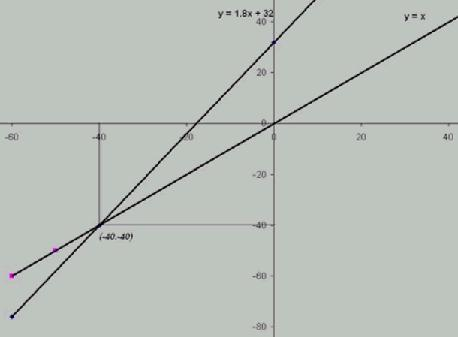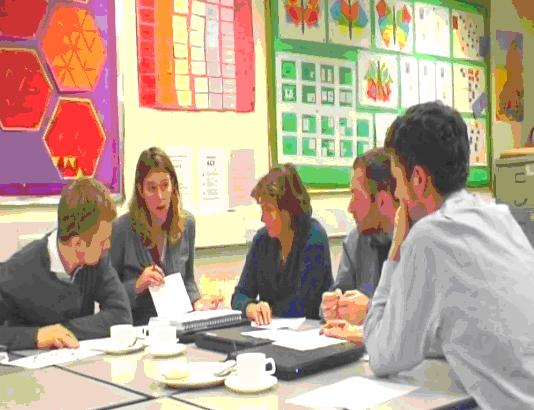# Optimising Input - Maximising Output

##### Age 11 to 18

Published February 2011.

A group of teachers involved in embedding NRICH tasks into their everyday practice decided they needed to address the (im)balance between teacher and student activity in their classrooms. In this article they share the issues they identified and what they have been doing to address them.

'We wanted to place a greater emphasis on lesson planning so that teachers and students could be more productive during lessons.'

We are using more of our time thinking about and preparing for lessons, and placing less emphasis on marking the output of the lessons. Preparation has focussed on how we can support students in tackling problems for themselves, rather than on demonstrating strategies for finding answers. The Teachers' Notes that accompany the NRICH problems have proved very helpful. See, for example, the Teachers' Notes for Triangles in Circles

How well are we doing?

Lessons were observed and evaluated by peers, with planning forming the main focus of discussion. The status of the planning process was raised by setting aside time for colleagues to work together on lesson preparation - this was effective use of teachers' limited time.

Teachers spent time thinking about how to present tasks that might maximise opportunities for students to develop their own ideas, including which key questions would be asked. The Teachers' Notes to the NRICH problems always list some key questions. See, for example, the Teachers' Notes for Reaction Timer http://nrich.maths.org/reactiontimer

Some schools are monitoring students' attitudes to the changing nature of lessons by surveying opinions.

Peer observation outcomes showed that staff valued an emphasis on planning.

Teachers are planning collaboratively and sharing ideas.

Teachers have begun to utilise Kenneth Ruthven's (1989) idea of: Exploration $\rightarrow$ codification $\rightarrow$ consolidation

'We wanted our students to take more responsibility for their learning.'

In our schools we have worked on establishing classroom communities of enquiry, where everyone's contributions are valued.

By setting aside a few minutes for students to consider, on their own, how they might approach new problems, schools are encouraging all students to become more resourceful and less dependent on others for guidance.

Students are encouraged to jot down notes as they think around problems, using mini-whiteboards or separate space set aside in exercise books for this purpose.

'We wanted the students and the teacher to work in a more active way.'

We have been encouraging students to present their findings and compare their results. They are encouraged to address their work to, and look for feedback from, the rest of the group, rather than just the teacher.

See, for example, the approach suggested in the Teachers' Notes to Peaches Today, Peaches Tomorrow...

How well are we doing?

Schools promoted active lessons by encouraging whole class discussions and/or small group discussions.

See, for example, the approach suggested in the Teachers' Notes to the Factors and Multiples Puzzle

Schools are using a range of evaluation methods including peer observation and student feedback to assess their progress towards this aim.

'We wanted to create lessons which are less dependent on talk led by the teacher, with more time spent by students discussing the ideas they are investigating.'

We have chosen rich tasks from the NRICH website http://nrich.maths.org which encourage discussion. For example

• Students have compared the efficiency of various strategies when working on questions that have been solved in a variety of ways. As well as comparing their own solutions, students also discuss published solutions from the NRICH website. See, for example, the range of published solutions to: Ben's Game, Temperature

The problem includes the following question: Is there a temperature at which Celsius and Fahrenheit readings are the same?

This is an extract from a solution sent in by Seb:

There is a temperature at which Celsius and Farenheit are the same. It is $-40$, because $\frac{9}{5}$ of $-40$ is $-72$ and $-72+32=-40$. I decided to look at negative numbers because starting with a positive number and multiplying it by is going to increase it and so is adding $32$ so you're always going to end up with a number greater than the number you started with. However, if you start with a negative number, multiplying it by decreases it, and adding $32$ increases it, so I realised that with the correct number, Celsius and Fahrenheit might be the same.

I decided to go down in tens: $\frac{9}{5}$ of $-10=-18$ and $-18+32=14$, so that doesn't work; $\frac{9}{5}$ of $-20=36$ and $-36+32=-4$, so that doesn't work; $\frac{9}{5}$ of $-30=-54$ and $-54+32=-22$, so that doesn't work But $\frac{9}{5}$ of $-40=-72$ and $-72+32=-40$ so it works.

The reason it works is because multiplying by $\frac{9}{5}$ is equivalent to adding $\frac{4}{5}$ of it, and for $-40$ adding $32$ is equivalent to subtracting $\frac{4}{5}$ of it (because $32$ is $\frac{4}{5}$ of $40$). Because of this, Farenheit and Celsius are equivalent ONLY at $-40$.

Alex, Ben, Chris and Paul also used a trial and improve approach:

First we started going down in tens of Celsius from $0$, and we found out a pattern: the difference between F and C was getting closer by eights every time. When we got to $-30$C the difference was only $8$. So $-30$C is equal to $-22$F. Then we tried $-40$C and found out that $-40$C was the same as $-40$F. So the answer is $-40$.

David used a graphical approach: I plotted the lines of the simultaneous equations against each other and found where they crossed. In the graphs $y=F$ and $x=C$.Phoebe offered an algebraic solution:

To solve it algebraically I can create two simultaneous equations: $$F=C$$ $$F=1.8C+32$$ Therefore $C=1.8C+32 C=\frac{32}{-0.8}=-40$

Schools have used the Curriculum Mapping documents published on the NRICH website to select suitable tasks which stimulate discussion.

How well are we doing?

Teachers encouraged students to develop convincing arguments by challenging them to: 'Convince yourself'' $\rightarrow$ 'Convince a friend' $\rightarrow$ 'Convince an enemy' as suggested by Mason et al. in Thinking Mathematically (Mason, Burton, Stacey 1982) One teacher encouraged discussion of findings by asking students to write down their solutions anonymously. These solutions were then used as a focus for review and comment by other students. Some schools reviewed the way they measured student performance in lessons. They not only considered how many questions had been answered correctly but also how students had reached their solutions and discussed their ideas. Rich tasks from the NRICH website have been embedded in curriculum documents and shared informally between colleagues.

'We wanted the students to focus on the mathematics, leaving the teacher free to focus on the students.'

Students have been learning to work independently whilst teachers have been learning to stand back. Teachers and students have begun to recognise that although it was frustrating when teachers withheld advice, it was a necessary part of learning to be a problem-solver.

Teachers have been able to give more of their attention to what students know, and this offers greater opportunities for identifying and addressing misconceptions.

'We wanted students to influence where problems lead.'During planning, we have tried to anticipate potential areas of further exploration. We have made use of solutions to problems on the NRICH site, which often give an insight into multiple routes to solutions and other areas for exploration. The Teachers' Notes to the NRICH problems also suggest possible extensions. See, for example, the Teachers' Notes to Square Coordinates, Pick's Theorem

How well are we doing?

Teachers have started to adopt flexible approaches which enable them to respond to the unexpected, such as students identifying a novel approach or different 'What if'?' questions.

In the most successful lessons students' questions have influenced the direction of the lesson.

'We wanted teachers and students to view the journeys to a solution as a valuable learning experience.'

We have focussed on listening carefully to students in order to draw out key ideas that could inform the next steps that students might choose to take.

We have often acted as if we are solving the problem alongside our students, sometimes acting as if the problem was new to us and sometimes discovering new directions on the way.

Our lessons have focussed more on the strategies being used, rather than just on reaching the answers. See, for example, Twisting and Turning

How well are we doing?

Teachers and students show interest in the strategies students adopt to reach their solutions.

Students and teachers are developing mathematical 'habits of mind' (Cuoco et al 1996)

References

Ruthven K (1989) An Exploratory Approach to Advanced Mathematics. Educational Studies in Mathematics 20: 449-467

Mason J with Burton L and Stacey K (1982) Thinking Mathematically, Addison Wesley Publishers Ltd.

Cuoco A , Goldenberg E P, Mark J (1996) Habits of Mind: An organising Principle for Mathematics Curricula. Journal of Mathematical Behaviour 15: 375-402